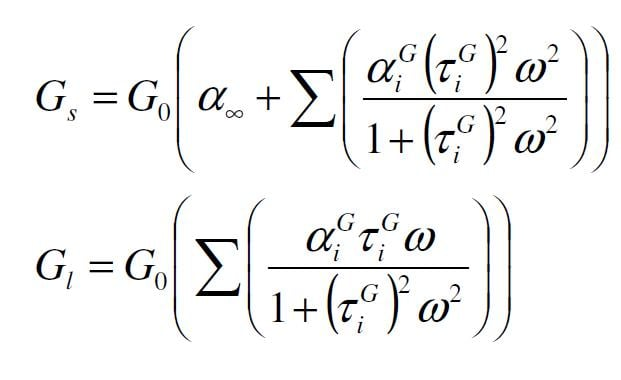## General Mechanical

•adkh
Subscriber
I want to perform modal analysis of viscoelastic sandwich structure, i.e, viscoelastic material in between two steel plates, in Ansys.
My question is that do i have to input only shear relaxation test data in ansys or i have to add additional data like uniaxial, planer, volumetric also for such type of analysis. Thanks
•Sandeep Medikonda
Ansys Employee
Hi Modal analysis is a linear analysis and non-linear materials or contacts cannot be used.

Regards Sandeep
•peteroznewman
Subscriber

Sandeep, I think adkh means vibration analysis when he says modal analysis, given the answers we gave in his other discussion.

•Sandeep Medikonda
Ansys Employee

ok thanks peter.

Yes, shear relaxation would do. You would only need bulk modulus if volumetric incompressibility is a factor. You might find this discussion helpful.

Regards,
Sandeep

•Bhargava Sista
Ansys Employee

Hi Sandeep, Peter,

Looking at this and the other discussion with adhk, I wanted to add something that might be relevant:

you can perform a full harmonic analysis on viscoelastic materials by defining frequency dependent elastic modulus, Poisson's ratio and damping using TB,ELASTIC and TB,DAMP commands (WB does not support this in GUI, so you'll need to use command snippets). It still assumes linear response so large-deformation effects are not included.

•adkh
Subscriber

Hi Sandeep, sorry i mean vibration analysis. Thanks peter for clarifying it.

•adkh
Subscriber

Hi bsista, can you please add a little more detail of your previous answer because i didn't understand, you mean that shear relaxation test w.r.t time should not be used?. Thanks

•Bhargava Sista
Ansys Employee

You can use the shear relaxation test to calculate the Prony series constants. These properties are in time-domain so you'll need to convert them into frequency-domain using the following equations, which is nothing but calculating the storage and the loss modulus.You'll need to define a starting and the ending frequency to calculate these quantities. Once you do this conversion, you can input these properties into ANSYS Mechanical using command snippets.

•adkh
Subscriber
Ok thank you so much, but what tool should i use to convert it from time domain to frequency domain, in case i have the data of relaxation test.
Secondly, can you provide a reason why i can't use directly the time domain data.
•Bhargava Sista
Ansys Employee

You can use ANSYS WB to perform curve fitting on shear relaxation data and calculate Prony series constants. Once you have them, you can use Matlab, Python, Excel or even APDL math (if you're familiar with it) to perform these conversions.

As Sandeep and Peter have mentioned earlier, viscoelasticity is a nonlinear material behavior while harmonic analysis is a linear analysis. So, we use Fourier transforms to linearize the property by converting the data from time-domain into the frequency domain.

•adkh
Subscriber

I understand now, thanks to Sandeep, Peter and Bsista.

•seventanapply
Subscriber

"Methods of interconversion between linear viscoelastic material functions Part I- a numerical method based on Prony series" by S W Park and R A Schapery.

•seventanapply
Subscriber

Hi bsista,

Is it possible to use transient structural module to see the phase lag angle delta?   tan delta is defined by loss modulus/storage modulus.

Thanks.

•JHPontes
Subscriber
Hi all,nI already have the data for storage and loss modulus vs frequency but not really sure how to insert it into ansys WB engineering data.nCould anyone help me?n
•Laurentiomoto
Subscriber
Im looking for the same answer as JHPontes .n